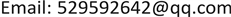1. 引言

2. 景德镇陶瓷产税收征管现状2.1. 陶瓷行业宏观税率偏低

2.2. 生产组织结构不完善

2.3. 税收文化发展的滞后

3. 博弈论分析

3.1. 基本假设

1) 理性假设  ：主体双方为税务机关和纳税人。首先，要承认税收征管中的征纳双方都是理性的，都要寻求自己利益实现极大化。对于纳税人来说，其目标是如何使自己在最小被发现的情况下(成本最小)，获取最多的逃税税款(只从单纯的经纪人角度分析，不考虑个体觉悟因素)。对于税务机关来说，其目标是依法正受到尽可能多的税款。但从经济学的角度以及税收的效率原则来看，税务机关的最终目的应该是以尽可能少的征管成本依法征收到尽可能多的税款，这样才能够说明税务机关的运行最有效率，因此，税务机关稽查的概率与其征管成本有关。

Comparison of ceramic production value and ceramics tax revenue in Jingdezhen 2011~2016 unit: 100 million yua

2011年192.63.0021.56
2012年2153.51.63
2013年249.33.861.55
2014年291.64.251.46
2015年336.54.381.30
2016年366.74.391.20

2) 资源有限假设：以征纳双方信息不对称为基本条件，税务部门的征管资源包括人、财、物等都是有限的，对其所管辖区域内的税收管理不可能将所有的事情都面面俱到，百无遗漏，在具体情况下或具体决策下税务机关只能将主要力量用于重点或指定选取的方面。

3) 可信度假定：税务机关是一支培训到位，有开展工作能力的队伍，只要有偷税行为的纳税人一经稽查便会受到处理。税务机关的征管人员行为是廉洁的。假设：

3.1) A = (d, e)代表纳税人的行动空间，其中d代表逃税的行为，e代表不逃税的行为。纳税人逃税的概率分布为P (A = d) = S，P (A = e) = 1 − S。

3.2) B = (f, h)是税务机关的行动空间，其中f代表稽查的行为，h代表不稽查的行为。税务机关稽查的概率分布为p (B = f) = G，P (B = h) = 1 − G。

3.2. 博弈模型的建立和分析

E ( 1,   S ) = S ( T − C + F ) + ( 1 − S ) ( T − C ) = SF + T − C E ( 0 , ​   S ) = S × 0 + ( 1 − S ) T = ( 1 − S ) T

E ( 1 ,   S ) = E ( 0 ,   S )

S = C / ( T + F )

Revenue matrix of tax authorities and taxpayer

E ( G,   1 ) = G ( H − F ) + ( 1 − G ) × ( H + T ) = − G ( T + F ) + H + T E ( G,   0 ) = GH + ( 1 − G ) H = H

E ( G,   1 ) = E ( G, ​   0 )

G = T / ( T + F )

4. 对于景德镇陶瓷税收征管的几点建议

4.1. 建立奖惩激励制度

4.2. 加强和完善纳税人纳税信息化建设

4.3. 优化陶瓷行业税收环境